Courses

# Test: Magnetism (Competition Level 2)

## 27 Questions MCQ Test Physics Class 12 | Test: Magnetism (Competition Level 2)

Description
This mock test of Test: Magnetism (Competition Level 2) for Class 12 helps you for every Class 12 entrance exam. This contains 27 Multiple Choice Questions for Class 12 Test: Magnetism (Competition Level 2) (mcq) to study with solutions a complete question bank. The solved questions answers in this Test: Magnetism (Competition Level 2) quiz give you a good mix of easy questions and tough questions. Class 12 students definitely take this Test: Magnetism (Competition Level 2) exercise for a better result in the exam. You can find other Test: Magnetism (Competition Level 2) extra questions, long questions & short questions for Class 12 on EduRev as well by searching above.
QUESTION: 1

### A current carrying wire is placed in the grooves of an insulating semi circular disc of radius `R', as shown. The current enters at point A and leaves from point B. Determine the magnetic field at point D.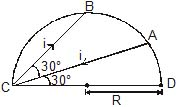Solution: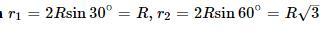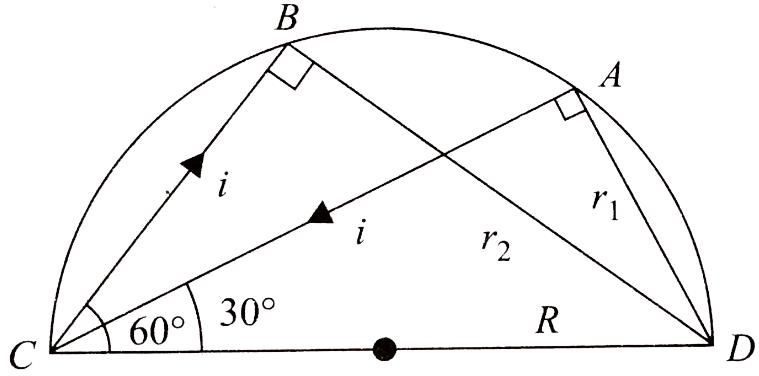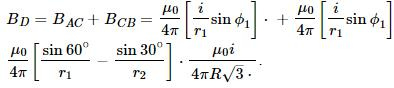QUESTION: 2

### A pair of stationary and infinitely long bent wires is placed in the X-Y plane as shown in figure. The wires carry currents of 10 A each as shown. The segments L and M are along thex-axis. The segments P and Q are parallel to the y-axis such that OS = OR = 0.02 m. Find the magnetic induction at the origin O.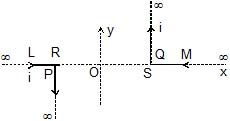Solution:

As point O is along the length of segments L and M, so the field at O due to these segments will be zero.
Also, point O is near one end of a long wire.
The resultant field at O, BR​=BP​+BQ​, this field will be into the plane paper.
⇒BR​= μ0I/4π​​RO​+​​ μ0I/4πSO​
But, RO=SO=0.02m
Hence, BR​=2× (μ0/4π) ​​×(10/0.02)=2×10−7 (10/0.02) ​=10−4Wb m−2

QUESTION: 3

### Determine the magniutde of magnetic field at the centre of the current carrying wire arrangement shown in the figure. The arrangement extends to infinity. (The wires joining the successive square are along the line passing through the centre)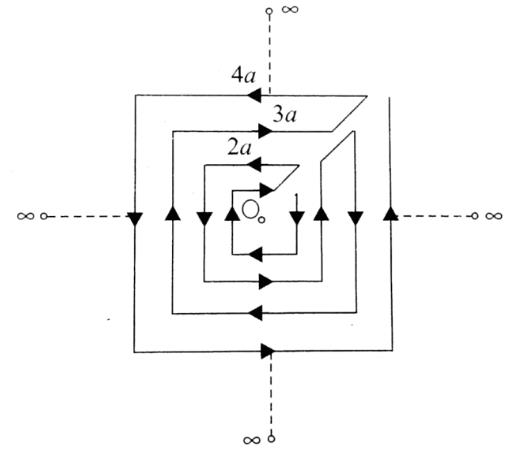Solution: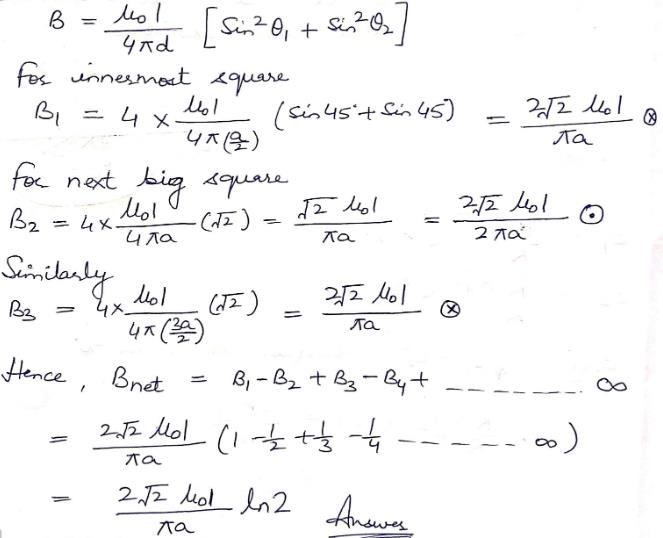QUESTION: 4

Figures shows a long wire bent at the middle to form a right angle. The magnitudes of the magnetic fields at the points P, Q, R and S are B1, B2, B3, B4respectively. The wire and the circumference of circle are coplaner. T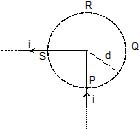Solution:
QUESTION: 5

Two long parallel wires situated at a distance 2a are carrying equal current `i' in opposite direction as shown in figure. The value of magnetic field at a point P situated at equal distances from both the wires will be :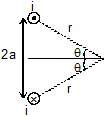Solution: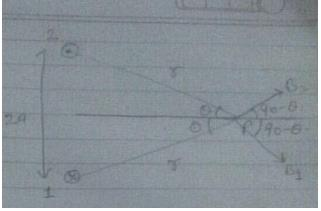We know that,
B1=B2μ0/2πr
Angle between B1 and B2
BR= √[B12+B22+2B1B2Cos(180-2θ)]
= √2B2+2B2(-Cos2θ)
=B√[2(1-Cos2θ)]
=B√[2(sin2θ)]
=2Bsina
B=(2μ0i/2πr) x (a/r)
B=(2μ0ia/2πr2)

QUESTION: 6

A uniform beam of positively charged particles is moving with a constant velocity parallel to another beam of negatively charged particles moving with the same velocity in opposite direction separated by a distance d. The variation of magnetic field B along a perpendicular line draw between the two beams is best represented by

Solution:

Currents are in the same direction, so at the middle B=D
And the B varies exponentially not linearly,
So,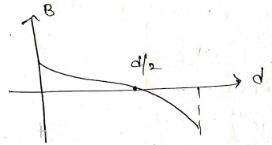QUESTION: 7

Infinite number of straight wires each carrying current I are equally placed as shown in the figure. Adjacent wires have current in opposite direction. Net magnetic field at point P is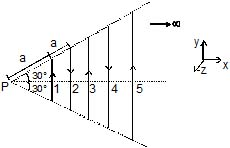Solution:

Magnetic field at P is equal to the magnetic field due to AB,CD,EF and so on.
Magnetic field due to AB is +Z direction.
and CD is negative −Z direction and EP is positive +Zdrection and so on.
BP​= (​μ0​I/4πd1)​ [sin30°−sin(−30°)k]+ ​(μ0​I/4πd2)​[sin30°−sin(−30°)l−k]+ μ0​I /4πd3​ ​[sin30°−sin(−30°)k]+...∞
BP​=2sin30°× (μ0​I​/4π) [(1/d1)​​−(1/d2​)​+(1/d3​)​−(1/d4​)​+(1/d5​)​....∞]k
= μ0​I/4π ​[(1/acos30°)​−(1/2acos30°)​+(1/3acos30°)−....∞]k
= μ0​I/4π(acos30°) ​[1−(1/2)​+(1/3)​−(1/4)​+(1/5)....∞]k
=(2μ0​I/4π√​3​a) ​ln(1+1)k= (μ0​I/4π√3​a) ​ln22k=(μ0​I/4π√3​a) ​ln4k

QUESTION: 8

Two mutually perpendicular conductors carrying currents I1 and I2 lie in one plane. Locus of the point at which the magnetic induction is zero, is a

Solution:

Magnetic field in X-Y plane at position (x,y) due to both wires must be zero
current carrying wire with current i1 is taken along X axis
now the magnetic field at the given point is
B1= μ0i1/2πy
now at the same point the magnetic field due to other wire placed along Y axis is given by,
B2= μ0i2/2πx
now we have to make the magnitude of magnetic field due to both wires same
so, B1=B2
μ0i1/2πy= μ0i2/2πx
now by solving above we have,
y=(i1/i2)x
so it’s a straight line with slope i1/i2 and passing from origin

*Multiple options can be correct
QUESTION: 9

Consider the magnetic field produced by a finitely long current carrying wire.

Solution:

If we take plane parallel to the length of wire function the all prints one plane will be current Field will be inversely proportional to the perpendicular distance from the wire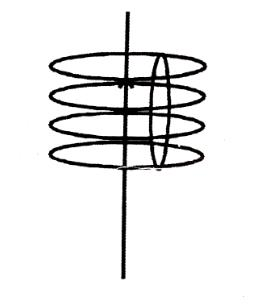*Multiple options can be correct
QUESTION: 10

Consider three quantities x =E/B, y =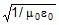and z =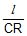. Here, l is the length of a wire, C is a capacitance and R is a resistance. All other symbols have standard meanings.

Solution:

As E=Bv, thus X has a dimension of velocity.
Also, c=1/μo​ϵo​​, where c is the speed of light.
So Y must have a dimension of velocity.
Now Z=l/CR​=l×(V/Q)​×(I/V)​=l×(1/Q)​×Q/t​=l/t​
Thus, Z also has a dimension of velocity.

*Multiple options can be correct
QUESTION: 11

Two long thin, parallel conductors carrying equal currents in the same direction are fixed parallel to the x–axis, one passing through y = a and the other through y = –a. The resultant magnetic field due to the two conductors at any point is B. Which of the following are correct ?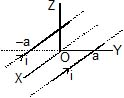Solution:

On x-axis
A.  B=(μ0I/2πa)- (μ0I/2πa)=0
B.  On y axis say at (y, 0, 0)
B=[-μ0I/2π(a+y)]k + [μ0I/2π(a-y)]k
So except at origin, B has only z-components.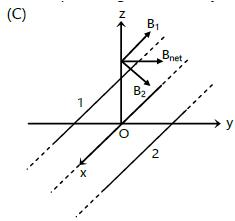B cannot have x-component as B is perpendicular to direction of I.

QUESTION: 12

Current flows through uniform, square frames as shown. In which case is the magnetic field at the centre of the frame not zero ?

Solution:

According to all figure given,
The magnetic field due to square shape in all options will be zero, Because distribution of current will be less in the ϕ longer path. But its cumulative effect of the magnetic field at the centre will be the same.

In option (A) and (B) current line is passing through the centre, So its perpendicular distance will be zero, so magnetic field in Both (A) and (B) will be zero due to incoming and outgoing current.
Refer image .1

Whereas in case of (D) Magnetic field due to incoming current and outgoing current will be equal and opposite so it will cancel out these effects.
Refer image .2
be in case of (C) Magnetic field will be in same direction with equal magnitude by the incoming and outgoing current, So its effect will not cancel
Option C.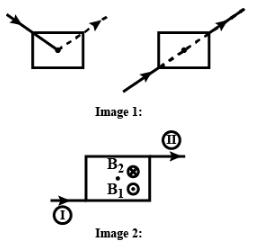QUESTION: 13

The two conductors of a transmission line carry equal current I in opposite directions. The force on each conductor is

Solution:
*Multiple options can be correct
QUESTION: 14

In the previous question, if the current is i and the magnetic field at D has magnitude B,

Solution:

Current around a long straight wire from Ampere's Law is given by-
B(2πr)=μ0​i
⟹B=μ0​i​/3πr
r is the distance of the point from the axis of the wire=√ (12+12​)=√2​
⟹B=μ0​i/2√2​π ​
The magnetic field is perpendicular to the vector pointing from the axis to the point at which the magnetic field is found.
The vector points from (1,0,0) to (1,1,1). Thus it makes angle 45oC with the xy plane.

QUESTION: 15

Three rings, each having equal radius R, are placed mutually perpendicular to each other and each having its centre at the origin of co-ordinate system. If current I is flowing through each ring then the magnitude of the magnetic field at the common centre is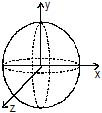Solution:

So, three rings each having equal radius R, are placed mutually perpendicular to each other and each having its centre at the origin of coordinate system If current I is flowing through each ring them the magnitude of the magnetic field at the common centre is:
Now,
we know,
B for circular loop = ui/2r. This formula is for the magnetic field at the centre of a circular loop of current.
So,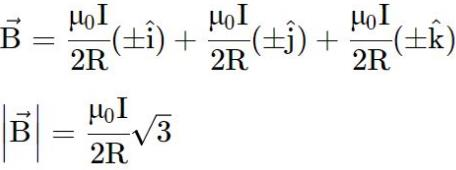QUESTION: 16

Two concentric coils X and Y of radii 16 cm and 10 cm lie in the same vertical plane containing N-S direction. X has 20 turns and carries 16 A. Y has 25 turns & carries 18 A. X has current in anticlockwise direction and Y has current in clockwise direction for an observer, looking at the coils facing the west. The magnitude of net magnetic field at their common centre is

Solution: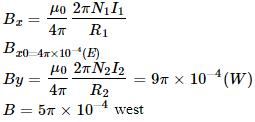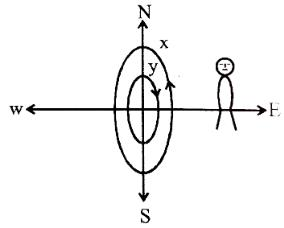QUESTION: 17

A current i is passed through a silver strip of width d and area of cross-section A. The number of free electrons per unit volume is n.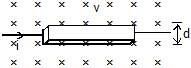Find the drift velocity v of the electrons.

Solution:

We know that,
I=V0nAe
V0=i/2ae

QUESTION: 18

A current i is passed through a silver strip of width d and area of cross-section A. The number of free electrons per unit volume is n.If a magnetic field B exists in the region as shown in figure, what is the average magnetic force on the free electrons ?

Solution:

F=q(VxB)
F=qVBsin θ                 (Here, θ=90o ,sinθ=1)
F=qVB                        (Here, V=I/nAe- drift velocity.)
F=ex (I/nAe) x B
F=IB/nA

QUESTION: 19

A current i is passed through a silver strip of width d and area of cross-section A. The number of free electrons per unit volume is n.Due to the magnetic force, the free electrons get accumulated on one side of the conductor along its length. This produces a transverse electric field in the conductor which opposes the magnetic force on the electrons. Find the magnitude of the electric field which will stop further accumulation of electrons.

Solution:
QUESTION: 20

A current i is passed through a silver strip of width d and area of cross-section A. The number of free electrons per unit volume is n.What will be the potential difference developed across the width of the conductor due to the electron accumulation ? The appearance of a transverse emf, when a current - carrying wire is placed in a magnetic field, is called Hall effect.

Solution:
*Answer can only contain numeric values
QUESTION: 21

Six wires of current I1 = 1 A, I2 = 2A, I3 = 3A, I4 = 1A, I5 = 5A and I6 = 4A cut the page perpendicularly at the points 1,2,3,4,5 and 6 respectively as shown in the figure. Find the value of the integral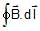around the closed path.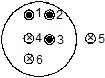Solution:

∫B.dl=µ0I(total current passing through the closed loop)
Currents outside the loop don’t contribute anything to the loop so I5 can be neglected, Also I4 and I6 are going into the loop.
=>∫B.dl= µ0(I1+I2+I3-I4-I6)
= µ0i wb/m

QUESTION: 22

Two circular coils of wire each having a radius of 4 cm and 10 turns have a common axis and are 6 cm apart. If a current of 1 A passes through each coil in the opposite direction find the magnetic induction.

Q.

At the centre of either coil

Solution:

I=1A, x=6x10-2  r=4x10-2m, N=10
B1= μ0​NIr2​/2(r2+x2)3/2
B1=2πx10-7x10x16x10-4/(16x10-4+36x10-4)3/2
B1=32x3.14x10-10/(52)3/2x10-6
B1=2.67x10-5T
Magnetic field due to another coil at centre is,
B2= μ0​NI/2r=2πx10-7x10/4x10-2
Since both are in opposite direction,
B=B2-B1
=(1.57-0.267)x10-4
B=1.3x10-4T

*Answer can only contain numeric values
QUESTION: 23

Two circular coils of wire each having a radius of 4 cm and 10 turns have a common axis and are 6 cm apart. If a current of 1 A passes through each coil in the opposite direction find the magnetic induction.

Q.

At a point on the axis, midway between them.

Solution:

We know Magnetic field at centre of a coil having Current I, radius r and number of turns N is given as B=μ0​NI​ /2r
and at a distance x from the centre and on the axis is B= [μ0​Nix] /[2(x2+r2)] ​ and its direction is given by right-hand screw rule i.e.
if the observer sees that the current is anticlockwise then the field will be towards the observer and vice-versa, as shown in the figure−1

Part a
Field at center O1​  will be difference of field due to loop-1 and loop-2
At O1​  Bnet​ =  (μ0​NI​/2r)  - [μ0​Nix]/[2(x2+r2)] ​ towards observer
putting μ0​=4π×10−7 ,N=6, r=.04 meter ,x= .06 meter and I = 1 all units in S.I. system
we get Bnet​=5.07×10−5 Tesla towards the observer
Similarly, At O1​ the filed due to circle 2 will dominate so net filed will be
Bnet​=5.07×10−5 Tesla in a direction away from the observer.

Part b
According to the above theory the field at the midpoint on the axis of joining the centres will be equal and opposite as current in loops is opposite so net field is zero there at O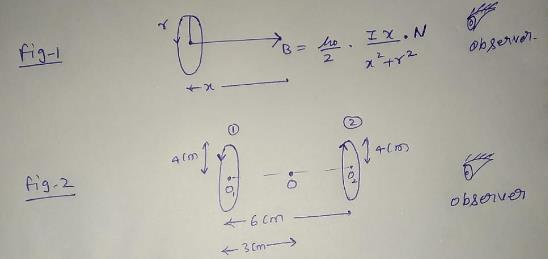QUESTION: 24

Find the ratio of magnetic field magnitudes at a distance 10 m along the axis and at 60° from the axis, from the centre of a coil of radius 1 cm, carrying a current 1 amp.

Solution:
QUESTION: 25

A particle of charge +q and mass m moving under the influence of a uniform electric field E and a magnetic field B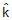enters in I quadrant of a coordinate system at a point (0, a) with initial velocity v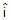and leaves the quadrant at a point (2a, 0) with velocity - 2v . Find

Magnitude of electric field

Solution:
QUESTION: 26

A particle of charge +q and mass m moving under the influence of a uniform electric field E and a magnetic field Benters in I quadrant of a coordinate system at a point (0, a) with initial velocity vand leaves the quadrant at a point (2a, 0) with velocity - 2v . Find

Rate of work done by the electric field at point (0, a)

Solution:

From the work- energy theorem
W=△K.E.
⇒qE.2a=(1/2)​m[4υ2−υ2]
⇒E=(3/4)​( mυ2​/qa)
At P rate of work done by E field will be
=F.υ=(qE)(υ)cos0°
=(3/4)​( mυ3/a​)

*Answer can only contain numeric values
QUESTION: 27

A particle of charge +q and mass m moving under the influence of a uniform electric field E and a magnetic field Benters in I quadrant of a coordinate system at a point (0, a) with initial velocity vand leaves the quadrant at a point (2a, 0) with velocity - 2v . Find

Rate of work done by both the fields at (2a, 0)

Solution:

From the work- energy theorem
W=△K.E.
⇒qE.2a=(1/2)​m[4υ2−υ2]
⇒E=(3/4)​( mυ2​/qa)
At P rate of work done by E field will be
=F.υ=(qE)(υ)cos0°
=(3/4)​( mυ3/a​)
At Q rate of work done
=F.υ=(qE)(2υ)cos90°=0
And work done by the magnetic field is always zero.